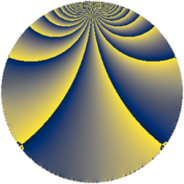# Properties

 Label 164.2.fLevel $164$ Weight $2$ Character orbit 164.f Rep. character $\chi_{164}(9,\cdot)$ Character field $\Q(\zeta_{4})$ Dimension $6$ Newform subspaces $1$ Sturm bound $42$ Trace bound $0$

# Related objects

## Defining parameters

 Level: $$N$$ $$=$$ $$164 = 2^{2} \cdot 41$$ Weight: $$k$$ $$=$$ $$2$$ Character orbit: $$[\chi]$$ $$=$$ 164.f (of order $$4$$ and degree $$2$$) Character conductor: $$\operatorname{cond}(\chi)$$ $$=$$ $$41$$ Character field: $$\Q(i)$$ Newform subspaces: $$1$$ Sturm bound: $$42$$ Trace bound: $$0$$

## Dimensions

The following table gives the dimensions of various subspaces of $$M_{2}(164, [\chi])$$.

Total New Old
Modular forms 48 6 42
Cusp forms 36 6 30
Eisenstein series 12 0 12

## Trace form

 $$6q + 2q^{3} + O(q^{10})$$ $$6q + 2q^{3} - 4q^{11} - 2q^{13} - 2q^{15} - 2q^{17} + 2q^{19} + 4q^{23} + 6q^{25} + 2q^{27} - 10q^{29} + 8q^{31} + 18q^{35} - 36q^{37} + 6q^{41} - 20q^{45} - 26q^{47} + 12q^{51} - 14q^{53} - 6q^{55} - 24q^{57} - 4q^{59} + 18q^{63} + 20q^{65} + 16q^{67} + 8q^{69} + 26q^{71} - 10q^{75} - 2q^{79} + 38q^{81} + 36q^{83} + 44q^{85} + 14q^{89} - 28q^{93} - 26q^{95} - 6q^{97} - 2q^{99} + O(q^{100})$$

## Decomposition of $$S_{2}^{\mathrm{new}}(164, [\chi])$$ into newform subspaces

Label Dim. $$A$$ Field CM Traces $q$-expansion
$$a_2$$ $$a_3$$ $$a_5$$ $$a_7$$
164.2.f.a $$6$$ $$1.310$$ 6.0.5089536.1 None $$0$$ $$2$$ $$0$$ $$0$$ $$q+\beta _{1}q^{3}+(-\beta _{4}+\beta _{5})q^{5}+(\beta _{1}+\beta _{2}+\cdots)q^{7}+\cdots$$

## Decomposition of $$S_{2}^{\mathrm{old}}(164, [\chi])$$ into lower level spaces

$$S_{2}^{\mathrm{old}}(164, [\chi]) \cong$$ $$S_{2}^{\mathrm{new}}(41, [\chi])$$$$^{\oplus 3}$$$$\oplus$$$$S_{2}^{\mathrm{new}}(82, [\chi])$$$$^{\oplus 2}$$